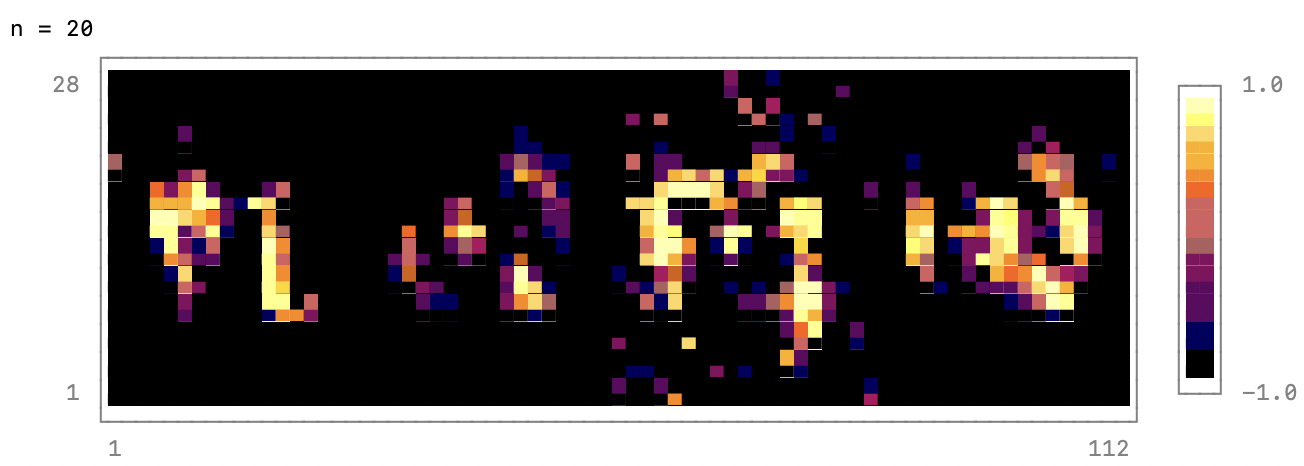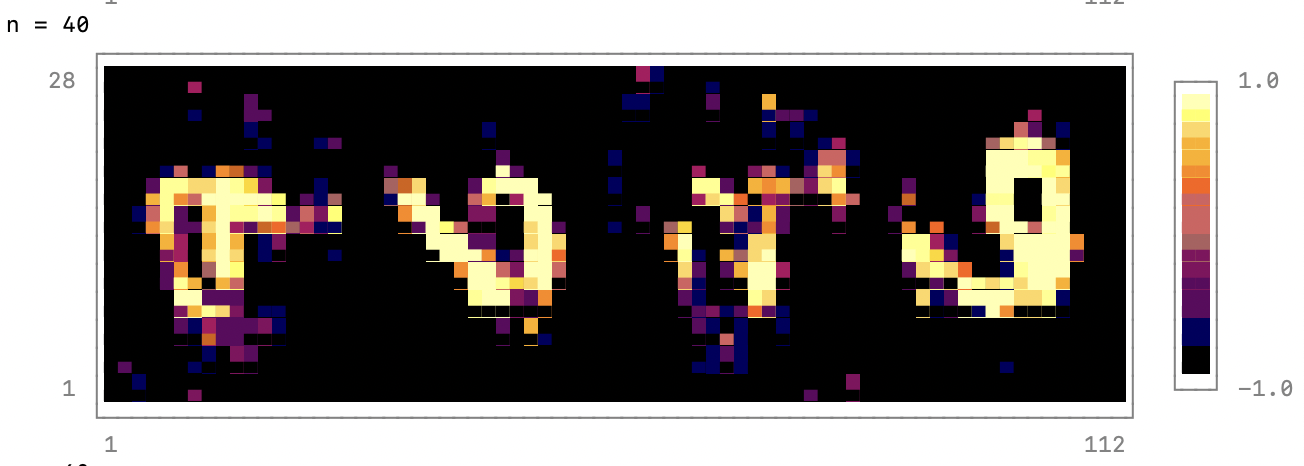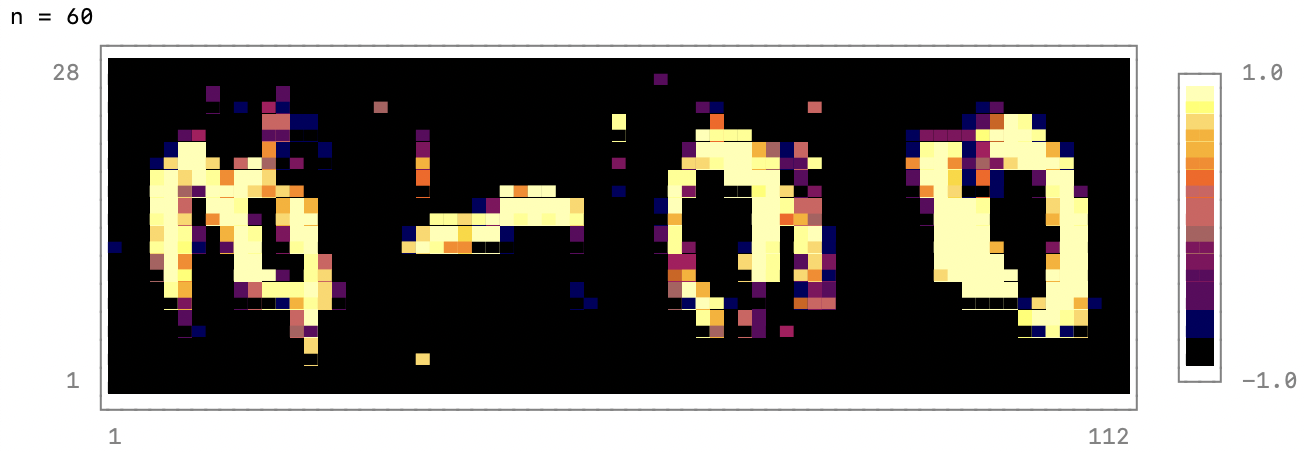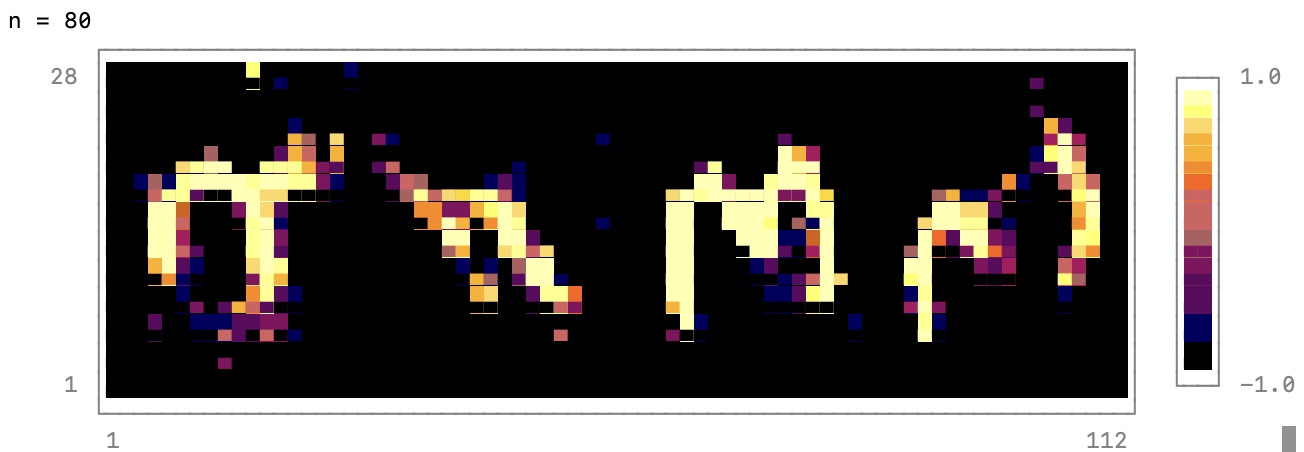# Generative Adversarial Networks

This tutorial describes how to implement a vanilla Generative Adversarial Network using Flux and how train it on the MNIST dataset. It is based on this Pytorch tutorial. The original GAN paper by Goodfellow et al. is a great resource that describes the motivation and theory behind GANs:

In the proposed adversarial nets framework, the generative model is pitted against an adversary: a
discriminative model that learns to determine whether a sample is from the model distribution or the
data distribution. The generative model can be thought of as analogous to a team of counterfeiters,
trying to produce fake currency and use it without detection, while the discriminative model is
analogous to the police, trying to detect the counterfeit currency. Competition in this game drives
both teams to improve their methods until the counterfeits are indistinguishable from the genuine
articles.


Let’s implement a GAN in Flux. To get started we first import a few useful packages:

using MLDatasets: MNIST
using Flux
using CUDA
using Zygote
using UnicodePlots


To download a package in the Julia REPL, type ] to enter package mode and then type add MLDatasets or perform this operation with the Pkg module like this

> import Pkg


While UnicodePlots is not necessary, it can be used to plot generated samples into the terminal during training. Having direct feedback, instead of looking at plots in a separate window, use fantastic for debugging.

Next, let us define values for learning rate, batch size, epochs, and other hyper-parameters. While we are at it, we also define optimizers for the generator and discriminator network. More on what these are later.

    lr_g = 2e-4          # Learning rate of the generator network
lr_d = 2e-4          # Learning rate of the discriminator network
batch_size = 128    # batch size
num_epochs = 1000   # Number of epochs to train for
output_period = 100 # Period length for plots of generator samples
n_features = 28 * 28# Number of pixels in each sample of the MNIST dataset
latent_dim = 100    # Dimension of latent space
opt_dscr = ADAM(lr_d)# Optimizer for the discriminator
opt_gen = ADAM(lr_g) # Optimizer for the generator


In this tutorial I’m assuming that a CUDA-enabled GPU is available on the system where the script is running. If this is not the case, simply remove the |>gpu decorators: piping.

The MNIST data set is available from MLDatasets. The first time you instantiate it you will be prompted if you want to download it. You should agree to this.

GANs can be trained unsupervised. Therefore only keep the images from the training set and discard the labels.

After we load the training data we re-scale the data from values in [0:1] to values in [-1:1]. GANs are notoriously tricky to train and this re-scaling is a recommended GAN hack. The re-scaled data is used to define a data loader which handles batching and shuffling the data.

    # Load the dataset
train_x, _ = MNIST.traindata(Float32);
# This dataset has pixel values ∈ [0:1]. Map these to [-1:1]
train_x = 2f0 * reshape(train_x, 28, 28, 1, :) .- 1f0 |>gpu;
# DataLoader allows to access data batch-wise and handles shuffling.


## Defining the Networks

A vanilla GAN, the discriminator and the generator are both plain, feed-forward multilayer perceptrons. We use leaky rectified linear units leakyrelu to ensure out model is non-linear.

Here, the coefficient α (in the leakyrelu below), is set to 0.2. Empirically,
this value allows for good training of the network (based on prior experiments). It has also been found that Dropout ensures a good generalization of the learned network, so we will use that below. Dropout is usually active when training a model and inactive in inference. Flux automatically sets the training mode when calling the model in a gradient context. As a final non-linearity, we use the sigmoid activation function.

discriminator = Chain(Dense(n_features, 1024, x -> leakyrelu(x, 0.2f0)),
Dropout(0.3),
Dense(1024, 512, x -> leakyrelu(x, 0.2f0)),
Dropout(0.3),
Dense(512, 256, x -> leakyrelu(x, 0.2f0)),
Dropout(0.3),
Dense(256, 1, sigmoid)) |> gpu


Let’s define the generator in a similar fashion. This network maps a latent variable (a variable that is not directly observed but instead inferred) to the image space and we set the input and output dimension accordingly. A tanh squashes the output of the final layer to values in [-1:1], the same range that we squashed the training data onto.

generator = Chain(Dense(latent_dim, 256, x -> leakyrelu(x, 0.2f0)),
Dense(256, 512, x -> leakyrelu(x, 0.2f0)),
Dense(512, 1024, x -> leakyrelu(x, 0.2f0)),
Dense(1024, n_features, tanh)) |> gpu


## Training functions for the networks

To train the discriminator, we present it with real data from the MNIST data set and with fake data and reward it by predicting the correct labels for each sample. The correct labels are of course 1 for in-distribution data and 0 for out-of-distribution data coming from the generator. Binary cross entropy is the loss function of choice. While the Flux documentation suggests to use Logit binary cross entropy, the GAN seems to be difficult to train with this loss function. This function returns the discriminator loss for logging purposes. We can calculate the loss in the same call as evaluating the pullback and resort to getting the pullback directly from Zygote instead of calling Flux.train! on the model. To calculate the gradients of the loss function with respect to the parameters of the discriminator we then only have to evaluate the pullback with a seed gradient of 1.0. These gradients are used to update the model parameters

function train_dscr!(discriminator, real_data, fake_data)
this_batch = size(real_data)[end] # Number of samples in the batch
# Concatenate real and fake data into one big vector
all_data = hcat(real_data, fake_data)

# Target vector for predictions: 1 for real data, 0 for fake data.
all_target = [ones(eltype(real_data), 1, this_batch) zeros(eltype(fake_data), 1, this_batch)] |> gpu;

ps = Flux.params(discriminator)
loss, pullback = Zygote.pullback(ps) do
preds = discriminator(all_data)
loss = Flux.Losses.binarycrossentropy(preds, all_target)
end
# To get the gradients we evaluate the pullback with 1.0 as a seed gradient.

# Update the parameters of the discriminator with the gradients we calculated above

return loss
end


Now we need to define a function to train the generator network. The job of the generator is to fool the discriminator so we reward the generator when the discriminator predicts a high probability for its samples to be real data. In the training function we first need to sample some noise, i.e. normally distributed data. This has to be done outside the pullback since we don’t want to get the gradients with respect to the noise, but to the generator parameters. Inside the pullback we need to first apply the generator to the noise since we will take the gradient with respect to the parameters of the generator. We also need to call the discriminator in order to evaluate the loss function inside the pullback. Here we need to remember to deactivate the dropout layers of the discriminator. We do this by setting the discriminator into test mode before the pullback. Immediately after the pullback we set it back into training mode. Then we evaluate the pullback, call it with a seed gradient of 1.0 as above, update the parameters of the generator network and return the loss.

function train_gen!(discriminator, generator)
# Sample noise
noise = randn(latent_dim, batch_size) |> gpu;

# Define parameters and get the pullback
ps = Flux.params(generator)
# Set discriminator into test mode to disable dropout layers
testmode!(discriminator)
# Evaluate the loss function while calculating the pullback. We get the loss for free
loss, back = Zygote.pullback(ps) do
preds = discriminator(generator(noise));
loss = Flux.Losses.binarycrossentropy(preds, 1.)
end
# Evaluate the pullback with a seed-gradient of 1.0 to get the gradients for
# the parameters of the generator
# Set discriminator back into automatic mode
trainmode!(discriminator, mode=:auto)
return loss
end


## Training

Now we are ready to train the GAN. In the training loop we keep track of the per-sample loss of the generator and the discriminator, where we use the batch loss returned by the two training functions defined above. In each epoch we iterate over the mini-batches given by the data loader. Only minimal data processing needs to be done before the training functions can be called.

lossvec_gen = zeros(num_epochs)
lossvec_dscr = zeros(num_epochs)

for n in 1:num_epochs
loss_sum_gen = 0.0f0
loss_sum_dscr = 0.0f0

for x in train_loader
# - Flatten the images from 28x28xbatchsize to 784xbatchsize
real_data = flatten(x);

# Train the discriminator
noise = randn(latent_dim, size(x)[end]) |> gpu
fake_data = generator(noise)
loss_dscr = train_dscr!(discriminator, real_data, fake_data)
loss_sum_dscr += loss_dscr

# Train the generator
loss_gen = train_gen!(discriminator, generator)
loss_sum_gen += loss_gen
end

# Add the per-sample loss of the generator and discriminator
lossvec_gen[n] = loss_sum_gen / size(train_x)[end]
lossvec_dscr[n] = loss_sum_dscr / size(train_x)[end]

if n % output_period == 0
@show n
noise = randn(latent_dim, 4) |> gpu;
fake_data = reshape(generator(noise), 28, 4*28);
p = heatmap(fake_data, colormap=:inferno)
print(p)
end
end


For the hyper-parameters shown in this example, the generator produces useful images after about 1000 epochs. And after about 5000 epochs the result look indistinguishable from real MNIST data. Using a Nvidia V100 GPU on a 2.7 GHz Power9 CPU with 32 hardware threads, training 100 epochs takes about 80 seconds when using the GPU. The GPU utilization is between 30 and 40%. To observe the network more frequently during training you can for example set output_period=20. Training the GAN using the CPU takes about 10 minutes per epoch and is not recommended.

## Results

Below you can see what some of the images output may look like after different numbers of epochs.